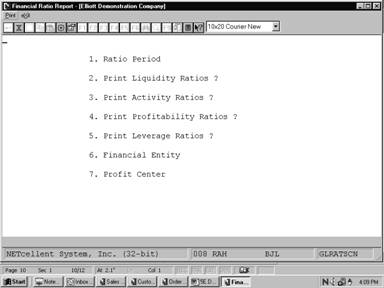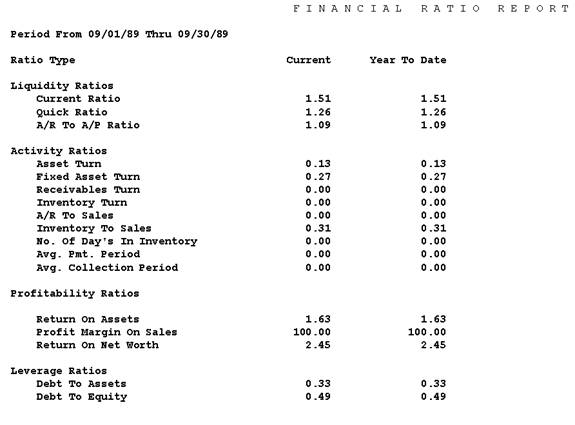General Ledger GLRATSCN Help - Elliott Business SoftwareElliott Home Elliott Business Software FAQ'S Add-ons Services 3rd Party Solutions News & Events Support Company Profile

Financial Ratio Report

## Financial Ratio Report

### Application Overview

The Financial Ratio Report provides a useful resource in both charting the performance of your organization and comparing it's various financial components over a given period of time. It displays four primary categories that measure business liquidity, activity, profitability, and leverage.  Each of these encompasses a group of ratios, derived from account information posted to the General Ledger File, according to the ratio type you set up in the G/L account file.

Before continuing further you should understand that even though ratio analysis is useful it has some limitations. Ratios are calculated with accounting data that can be affected by such variables as methods of depreciation and inventory validation. The interpretation of this information can vary among firms using different accounting procedures. You might want to seek the advice of a financial consultant in clarifying the trends suggested by this report.

Note:  Due to the nature of the Ratio Calculation, both Year To Date and Current Period values will be calculated using the same data.  The calculation for Gross Profit on Sales will be the only ratio calculated differently for the Year To Date and Current Periods.

#### Calculation of Ratio's

##### Averages

Averages are used to calculate Activity Ratios, and affect Balance Sheet type accounts.

The calculation used to determine the average for one of these values is defined as follows:

Average Value = (Ending Balance for the Last Fiscal Year + the sum of all of the Ending Balances for the periods up to the Current Period) divided by the number of periods being evaluated plus one.  The denominator or periods are incremented by one to account for the Ending Balance for the Last Fiscal Year added to the numerator.

By using this type of a calculation it is estimated that a more accurate average can be calculated.  For example, a calculation to determine an average Accounts Receivable Balance for period 3 would be calculated as follows:

(BBF+EB1+EB2+EB3)/4

BBF      =          The Ending Value of the Last Fiscal Year.

EB1      =          The Ending Balance of the 1st. Period

EB2      =          The Ending Balance of the 2nd. Period

EB3      =          The Ending Balance of the 3rd. Period

Example

Acct No: 01040-00000-00000    Accounts Receivable

BBF             EB1             EB2             EB3             Total           Average

10,000          11,000          15,000          19,000          55,000          13,750

##### Annualization

An annualization of a balance is used for P&L type Accounts and BSNI (Net Income).  It is used to forecast an annual value based on the known activity through the current period being evaluated.

The calculation to be used to estimate the Annualized amount is calculated as follows;

[EB(CP)/CP] x (No. of Periods)

The Ending Balance for the Current Period divided by the period number that you are in multiplied by the number of Periods in the Period Account File.

EB(CP) = The Ending Balance for the Current Period.

CP = Current Period Number

No. of Periods = The number of periods that are in the Period Account File.

From a statistical standpoint, using an annualized amount for ratio calculations generates more meaningful ratio data throughout the year.  This Ratio data should be considered an interim value of the projected Year End performance.

Example

Assuming that the Period Account File has 12 Periods and the Current Period is Period Number 3.

Sales:

EB3             Annualized Amount

250,000         1,000,000

#### Ratio Categories

There are four groupings of ratios displayed.  They include Liquidity, Activity, Profitability, and Leverage ratios.

#### Liquidity Ratio Types

Liquidity ratios measure your organization's capability of meeting its short term obligations. For example if your company has a sufficient amount of liquidity, it can take advantage of new investment opportunities and respond to a crisis.

 Ratio Description and Formula Current Ratio Measures your organization's ability to pay it's current liabilities from cash, cash equivalents and inventory.   Current Ratio              =              CA/CL   CA                           =              Current Assets CL                            =              Current Liabilities   Current Assets CA are equal to the sum of the Ending Balance for the Current Period for Cash C + Receivables R + Inventory I + Other Current Assets CA.   Current Liabilities CL are equal to the sum of the Ending Balance for the Current Period for Payables P + Other Current Liabilities CL. Quick Ratio This is a more rigid measurement of liquidity than the current ratio. It measures your ability to pay current liabilities with cash and receivables or "Liquid" cash equivalents.   Quick Ratio =  (C + R + CA)/ CL.   These values are equal to the Ending Balance for the Current Period for C              =              Cash R              =              Receivables CA           =              Other Current Assets CL            =              Current Liabilities. A/R To A/P Ratio Indicates whether your company is receiving more money than it is paying out.   A/R To A/P Ratio = R/P .   These values are equal to the Ending Balance for the Current Period for:   R              =              Receivables P              =              Payables.

#### Activity Ratio Types

Activity ratios can be considered as a gauge that measures the amount invested in assets by your business relative to the revenue generated by those assets.

 Ratio Description and Formula Asset Turn Calculates how effectively your business is employing its total assets to generate sales.    The Asset Turn Ratio = S/TA    S              =              Sales (annualized) TA            =              Total Assets   The Sales S value is annualized.  For a complete definition and calculation of annualization, see the Application Overview.

#### Profitability Ratio Types

Profitability Ratios measure your rate of success in generating sales over a given period of time.

 Ratio Description and Formula Return On Assets Ratio This ratio is also termed the rate of return on investment. It measures your organization's net income, to total asset investment.   Return On Assets Ratio = BSNI/TA   BSNI        =              Balance Sheet Net Income (annualized) TA            =              Total Assets   The BSNI value is annualized.  For a complete definition and calculation of annualization, see the Application Overview.   Total Assets TA is the sum of the Ending Balance for the Current Period for Cash C + Receivables R + Inventory I + Current Assets CA + Property, Plant & Equipment E - Depreciation D + Other Non-Current Assets OA. Profit Margin On Sales Ratio Enables you to discern the percentage of gross profit for each dollar of sales.   Profit Margin On Sales Ratio = (S - CS)/S   These values will use both the period net change value and the Ending Balance value to generate both a Current Period Ratio and a YTD Ratio using the following   S              =              Sales CS           =              Cost Of Goods Sold. Return On Net Worth Ratio This ratio measures your firms net income to its total net worth.   Return On Net Worth Ratio = BSNI/(TA - TL)   BSNI        =              Balance Sheet Net Income  (annualized) TA            =              Total Assets TL             =              Total Liabilities Return On Net Worth Ratio (continued) The BSNI value is annualized.  For a complete definition and calculation of annualization, see the Application Overview.   Total Assets TA is the sum of the Ending Balance for the Current Period for Cash C + Receivables R + Inventory I + Current Assets CA + Property, Plant & Equipment E - Depreciation D + Other Non-Current Assets OA.    Total Liabilities TL is the sum of the Ending Balance for the Current Period for Payables P + Other Current Liabilities CL + Long Term Liability OL.

#### Financial Leverage Ratio Types

Financial Leverage ratios measure the amount of financial burden placed on your business by it's debts.

 Ratio Description and Formula Debts To Assets Ratio Calculates the percentage of your total assets that are financed with creditors money.    The Debt To Asset Ratio = TL/TA   TL             =              Total Liability TA            =              Total Assets   Total Assets TA is the sum of the Ending Balance for the Current Period for Cash C + Receivables R + Inventory I + Current Assets CA + Property, Plant & Equipment E - Depreciation D + Other Non-Current Assets OA.    Total Liabilities TL is the sum of the Ending Balance for the Current Period for Payables P + Other Current Liabilities CL + Long Term Liability OL. Debt To Equity Ratio Contrasts your firm's total liabilities to the amount of equity.   Debt To Equity Ratio = TL/EQ   TL             =              Total Liability EQ           =              Equity   Total Liabilities TL is the sum of the Ending Balance for the Current Period for  Payables P + Other Current Liabilities CL + Long Term Liability OL.   Equity EQ is the sum of the Ending Balance for the Current Period for:   Retained earnings RE + Common/Preferred stock ST + Other Equity OE + BSNI.

### Run Instructions

Select Financial Ratio Report from the pull down G/L Reports window.  The following screen will then be displayed:Financial Ratio Report Entry Screen

The following options are available:

*           Select the desired mode from the Financial Ratio Report menu bar

*           Enter the data requested on the screen

To return to the menu bar, press the ESC or F10 key. To leave this application, press X for EXIT when positioned at the menu bar.

#### Entry Field Descriptions

 Name Type and Description 1.  Ratio Period 2 numeric characters.  Enter the period number that you want considered in the report. This number must be on file in the Account Period File application. 2. Print Liquidity  Ratios? Y or N.  Enter Y to display liquidity ratios or N to decline. This field defaults to Y. 3.  Print Activity  Ratios? Y or N.  Enter Y to print activity ratios or N to decline. This field defaults to Y. 4.  Print Profitability  Ratios? Y or N.  Enter Y to print profitability ratios or N to decline. This field defaults to Y. 5.  Print Leverage   Ratios Y or N.  Enter Y to print leverage ratios or N to decline.  This Field defaults to N. 6.  Financial Entity 5 alphanumeric characters.  Enter a valid financial entity number present in your Financial Entity File.  The entity description will then automatically display.   If you want to skip this field, press RETURN. 7.  Profit Center A standard profit center number in the standard profit center number format.   If your company does not use profit center, this field will be skipped.  Press RETURN to default to All profit center.   If a non-blank financial entity was entered above, the entry of this field will be skipped.Financial Ratio Report